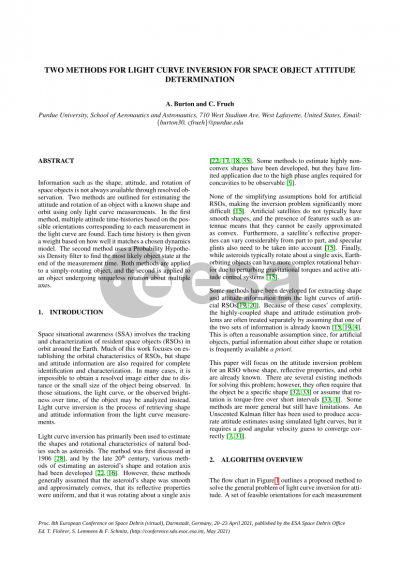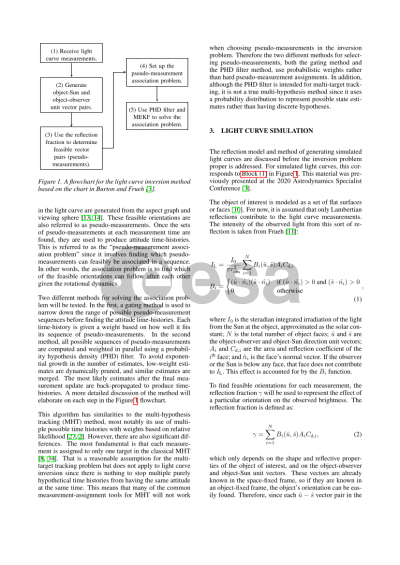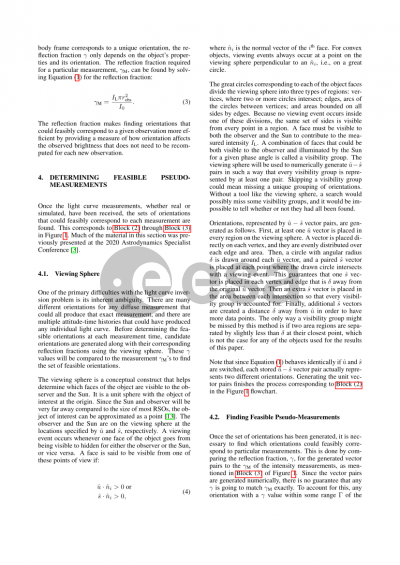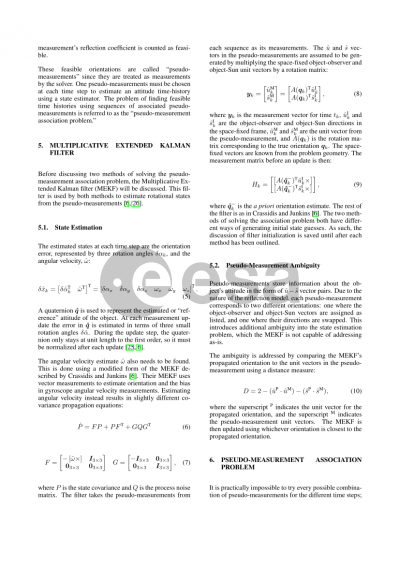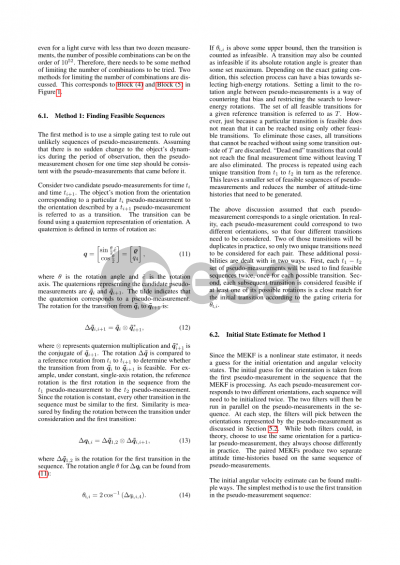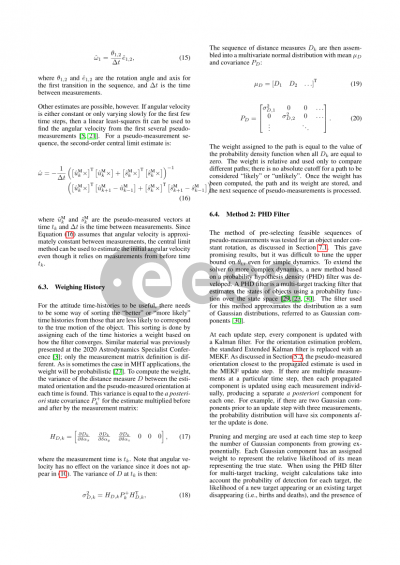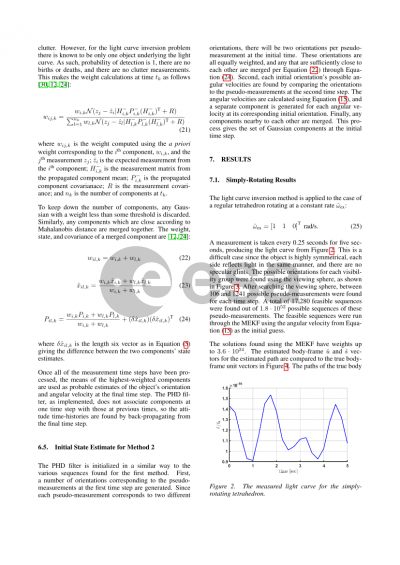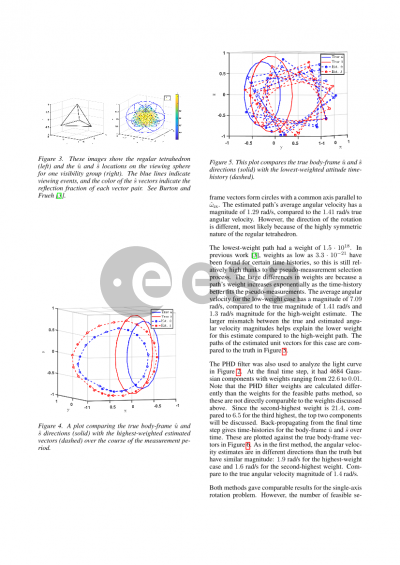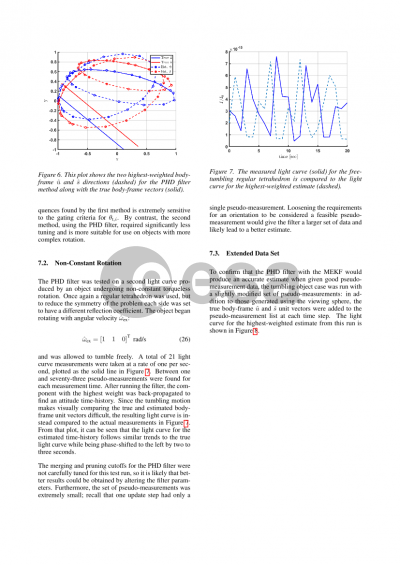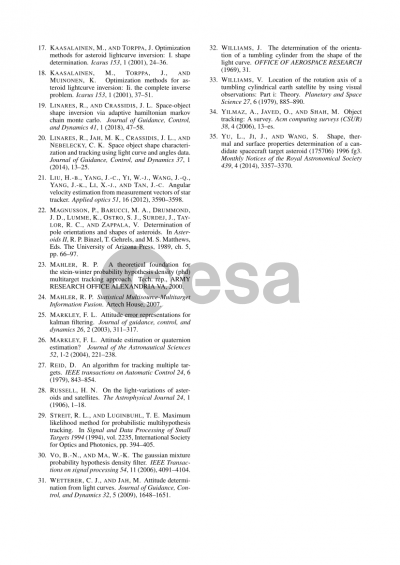# Multi-Hypothesis Light Curve Inversion for Space Object Attitude Determination

Alexander Burton1,Carolin Frueh1
Purdue University1

## Document details

Publishing year2021 PublisherESA Space Debris Office Publishing typeConference Name of conference8th European Conference on Space Debris
Pagesn/a Volume
8
Issue
1
Editors
T. Flohrer, S. Lemmens, F. Schmitz
Download paper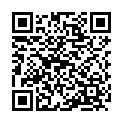## Abstract

Complete characterization of an object in space frequently requires knowing its attitude over time. However, in many cases, it is impossible to obtain a resolved image of the object so that its attitude would be directly observed. In such cases, analysis of light curve measurements can be an effective way to estimate the object's rotation. However, the light curve inversion problem does contain ambiguities.

Several methods already exist for carrying out this analysis, but they often rely on specific assumptions about the type of rotation that the object is undergoing. One common assumption is that the rotation is approximately stable, i.e., it does not change significantly over short periods. This paper presents a multi-hypothesis method for estimating an object's attitude time history that does not rely intrinsically on any prior assumptions about its rotation. The object's shape and reflective properties are assumed to be known.

This is an effective method, but there are also difficulties caused by the problem's inherent ambiguity. Each brightness measurement could correspond to many different object orientations. The viewing sphere concept is used to find a number of possible orientations for each measurement in the light curve to account for this ambiguity. The viewing sphere is a conceptual tool used to categorize which faces of a shape are visible to an observer as its orientation changes. The possible orientations found this way are referred to as "pseudo-measurements''. An attitude time-history can be constructed by selecting one attitude pseudo-measurement at each time step then using a multiplicative extended Kalman filter (MEKF) to estimate the orientation and angular velocity. The MEKF can be made to work with any dynamics model.

The number of possible combinations of pseudo-measurements can be very large, even for a small number of measurements. A gating system is used to narrow the range of possible combinations by finding feasible sequences of pseudo-measurements for a given dynamics model. First, the rotation from each pseudo-measurement at one time step to each pseudo-measurement at the next time step is found. Then, the rotations are compared to the expected rotation for that time step. This expected rotation is based on the dynamics chosen for the problem. If the rotation to go from one pseudo-measurement to another is not close to the expected rotation, then that transition is considered infeasible. Once all of the feasible transitions from one measurement time to another have been found, all the possible chains of feasible transitions from the initial measurement time to the final time step are found and stored. This gives a condensed list of pseudo-measurement sequences that are used by the MEKF to produce attitude time-histories.

Even with the smaller set of pseudo-measurement combinations selected via gating, there will still be a large number of candidate attitude time-histories for the object. A weighting measure is used to select the most likely candidate. Each attitude time-history is given a weight based on how well the MEKF estimated attitude matches the pseudo-measurement at each time step. Low-scoring time histories are assumed to be less likely than high-scoring ones. The method will be demonstrated by assuming different attitude profiles with simulated light curve data where the object's true state is known.

## Preview